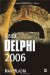Overloading an operator in Delphi is as simple as creating a function. The only difference is that instead of the reserved word function, you have to use the reserved words class and operator in the following way:

type   TMyRecordOrClass = record|class     class operator OperatorName(RequiredParameters): ResultType;   end;

The following table shows several operators that can be overloaded in Delphi. To see a complete list of operators that can be overloaded in Delphi, open Delphi's Help and load the "Operator Overloading" topic (ms-help://borland.bds4/bds4ref/html/OperatorOverloads.htm).

Listing 30-9 shows how to overload the Explicit and Add operators in a record.

Table 30-1: Several overloadable operators in Delphi

Operator

Operator Syntax

Explicit

Explicit(A: SrcType): ResultType

(Explicit Typecast)

Equal

Equal(A: Type; B: Type): ResultType

=

+program Project1; {\$APPTYPE CONSOLE} uses Types;        // for TRect type type   TMyPoint = record   public     X: Integer;     Y: Integer;     constructor Create(AX, AY: Integer);     class operator Add(A, B: TMyPoint): TMyPoint;     class operator Explicit(A: TRect): TMyPoint;   end; constructor TMyPoint.Create(AX, AY: Integer); begin   X := AX;   Y := AY; end; class operator TMyPoint.Add(A, B: TMyPoint): TMyPoint; begin   Result.X := A.X + B.X;   Result.Y := A.Y + B.Y; end; class operator TMyPoint.Explicit(A: TRect): TMyPoint; begin   Result.X := A.Left;   Result.Y := A.Top; end; var   RecOne, RecTwo: TMyPoint;   RecResult: TMyPoint;   Exp: TMyPoint;   RC: Types.TRect; begin   RecOne := TMyPoint.Create(10, 20);   RecTwo := TMyPoint.Create(100, 200);   RecResult := RecOne + RecTwo;   WriteLn(RecResult.X);      // 110   RC := Rect(1, 1, 10, 10);   // without the overloaded Explicit operator,   // this typcast would produce a compiler error   Exp := TMyPoint(RC);   WriteLn(Exp.X);           // 1   ReadLn; end.When overloading operators in C#, you need to remember that all operator overloads must be both public and static. You should also notice that there are syntactical differences between an operator and a conversion overload. Here's the syntax for overloading operators and the explicit typecast:

public static ReturnType operator Symbol(Type a, Type b) { } public static explicit operator ReturnType(SourceType) { }

Listing 30-10 shows how to overload the + operator and explicit typecast. It is the C# version of the Delphi example in Listing 30-9.using System; namespace Wordware.OperatorOverloading {    struct Rect    {       public int Left;       public int Top;       public int Right;       public int Bottom;       public Rect(int newLeft, int newTop,         int newRight, int newBottom)       {         Left = newLeft;         Top = newTop;         Right = newRight;         Bottom = newBottom;       }    }    struct MyPoint    {       public int X;       public int Y;       public MyPoint(int newX, int newY)       {         X = newX;         Y = newY;       }       // + operator overload       public static MyPoint operator + (MyPoint a, MyPoint b)       {         return new MyPoint(a.X + b.X, a.Y + b.Y);       }       // explicit typecast overload       public static explicit operator MyPoint(Rect a)       {         return new MyPoint(a.Left, a.Top);       }    }    class OverloadUser    {        [STAThread]       static void Main(string[] args)       {         MyPoint RecOne = new MyPoint(10, 20);         MyPoint RecTwo = new MyPoint(100, 200);         MyPoint RecResult = RecOne + RecTwo;   // + overload         Console.WriteLine(RecResult.X);        // 110         Rect RC = new Rect(1, 1, 10, 10);         MyPoint Exp = (MyPoint)RC;            // explicit overload         Console.WriteLine(Exp.X);             // 1         Console.ReadLine();       }    } }Inside Delphi 2006 (Wordware Delphi Developers Library)
ISBN: 1598220039
EAN: 2147483647
Year: 2004
Pages: 212